Simple linear regression using R2OpenBUGS

Yo dawg! I heard you like reproducible research,
so I put some knitr and R markdown in your R2OpenBUGS tutorial
so you can try out the code while you read about it!

Here we're going to look at using OpenBUGS and the R2OpenBUGS package in R to perform some simple linear regression. The model itself is so simple it's trivial, the main aim here is to concentrate on the R functions and what BUGS returns.

``````library(R2OpenBUGS)
``````

Next, write the model as though it was an R function. Note that the function does not have any arguments. Syntax used here is BUGS syntax. The function will be translated into BUGS code, so it's important to retain appropriate syntax for BUGS. If using constraints on parameters e.g. “I(0,)” then the syntax differs from the standard BUGS syntax - see the later example (Alternative 4). Note that in BUGS the ordering of likelihood and priors does not matter. The fact that we use alpha and beta before defining the prior does not matter. The convention is to specify the likelihood first and priors second.

``````
linemodel <- function() {
for (j in 1:N) {
Y[j] ~ dnorm(mu[j], tau)  ## Response values Y are Normally distributed
mu[j] <- alpha + beta * (x[j] - xbar)  ## linear model with x values centred
}
## Priors
alpha ~ dnorm(0, 0.001)
beta ~ dnorm(0, 0.001)
tau ~ dgamma(0.001, 0.001)
sigma <- 1/sqrt(tau)
}
``````

Next, let's prepare the data for analysis. BUGS accepts named list format data, including data structures with named columns (or mixtures of these). Note that BUGS doesn't react well if data passed to it is not used in the model… The best advice is to only pass what you need. Fortunately R is the perfect tool for preparing data for BUGS.

``````linedata <- list(Y = c(1, 3, 3, 3, 5), x = c(1, 2, 3, 4, 5), N = 5, xbar = 3)
``````

The next step is to provide initial values for the MCMC. You should provide initial values for all random variables in the model. If you do not, BUGS will attempt to derive initial values for any random variables that are not initialised by using what you have provided. However sometimes it is unable to do so. The initial values are passed as a function with no arguments containing a named list with names corresponding to each random variable in the MCMC. R2OpenBUGS will call this function for every MCMC chain that is specified, generating initial values for each chain. Here we have integer values for alpha and beta. As we are running only 1 MCMC chain this is acceptable, but if we were running >1 chain, each chain would have the same starting values (which is not ideal!)

``````lineinits <- function() {
list(alpha = 1, beta = 1, tau = 1)
}
``````

Finally, let's run BUGS using the bugs(…) command and assign the output to an object called “lineout”. Note that we need to specify which nodes/parameters (random variables) in the model to save at each step of the MCMC. Basically, anything except data can be monitored by BUGS - model parameters, missing data values, functions of model parameters… This is a strength of BUGS since all of the parameters will have their posterior distribution samples saved for summarising, graphing etc. It can also place an overhead in data handling however: saving 1000 MCMC samples x k chains for elements such as fitted values e.g. mu[j] above can lead to a lot of data values. In this case it is trivial, but for other, larger datasets it can lead to problems.

Note also that here we only specify the number of MCMC iterations to be performed. By default R2OpenBUGS assumes that half of these iterations will be used as the burn-in. So in this instance the posterior distribution will be based on a sample of 500 MCMC iterations. In the case of this linear model that is probably sufficient - but also the number of iterations used as burn-in here is probably overkill.

``````## Uses default settings for n.burnin = n.iter/2; n.thin=1;
lineout <- bugs(data = linedata, inits = lineinits, parameters.to.save = c("alpha",
"beta", "sigma"), model.file = linemodel, n.chains = 1, n.iter = 10000)
``````

Now we can examine the lineout object.

``````lineout
``````
``````## Inference for Bugs model at "C:/DOCUME~1/Owner/LOCALS~1/Temp/RtmpUlVF9i/modelb2c5027762b.txt",
## Current: 1 chains, each with 10000 iterations (first 5000 discarded)
## Cumulative: n.sims = 5000 iterations saved
##          mean  sd 2.5%  25%  50%  75% 97.5%
## alpha     3.0 0.6  1.9  2.7  3.0  3.3   4.1
## beta      0.8 0.4  0.1  0.6  0.8  1.0   1.6
## sigma     1.0 0.7  0.4  0.6  0.8  1.2   2.8
## deviance 13.0 3.7  8.8 10.2 12.0 14.6  22.9
##
## DIC info (using the rule, pD = Dbar-Dhat)
## pD = 3.9 and DIC = 16.9
## DIC is an estimate of expected predictive error (lower deviance is better).
``````

Let's also look at the attributes of this object:

``````attributes(lineout)
``````
``````## \$names
##   "n.chains"        "n.iter"          "n.burnin"
##   "n.thin"          "n.keep"          "n.sims"
##   "sims.array"      "sims.list"       "sims.matrix"
##  "summary"         "mean"            "sd"
##  "median"          "root.short"      "long.short"
##  "dimension.short" "indexes.short"   "last.values"
##  "isDIC"           "DICbyR"          "pD"
##  "DIC"             "model.file"
##
## \$class
##  "bugs"
``````

We'll take a closer look at the lineout object and its various components in the later example.

Alternative 1

Now let's repeat the same example, but this time run 3 MCMC chains. To do this we only need to change the inits(…) function that we specified previously. We do this by again specifying a function called “lineinits” but this time we assign a random sampling function for each of the named random variables in the list. Here we are using random Normal samples for “alpha” and “beta” and a random Uniform sample for “tau”. The Uniform distribution ensures that tau is not negative.

``````## Set initial values for many chains using functions like rnorm or runif
## Each chain draws a new starting value from the distributions
lineinits <- function() {
list(alpha = rnorm(1, 0, 1), beta = rnorm(1, 0, 1), tau = runif(1, 0, 1))
}
``````

Now when we run BUGS we specify 3 chains. Note here we specify the number of burn-in iterations explicitly: 100. This means that, with 3 MCMC chains, we will have posterior samples based on 3 x 900 iterations = 2700 iterations. Posterior distributions and summaries pool samples across chains by default.

``````## n.iter, n.burnin, n.thin dictate precisely how many iterations to do
## n.iter includes burnin
lineout <- bugs(linedata, lineinits, c("alpha", "beta", "sigma"), linemodel,
n.iter = 1000, n.burnin = 100, n.thin = 1, n.chains = 3)
``````

Let's look at the summary statistics of that run:

``````lineout\$summary
``````
``````##             mean     sd    2.5%     25%     50%     75%  97.5%  Rhat n.eff
## alpha     2.9965 0.5293 1.99938  2.7480  3.0045  3.2523  3.984 1.004  2700
## beta      0.7994 0.3968 0.07003  0.6308  0.8003  0.9722  1.507 1.000  2700
## sigma     0.9931 0.6596 0.41463  0.6235  0.8285  1.1430  2.591 1.001  2700
## deviance 12.8095 3.5627 8.78042 10.1900 11.9500 14.3700 21.623 1.001  2700
``````

We can also plot the lineout object, although the default visualisation isn't immediately useful. It shows the 80% percentiles for the monitored nodes across the 3 chains. This is a quick test of convergence of the MCMC chains. If the 3 intervals overlap it increases our confidence of the chains converging to the same final solution.

``````## Summary plot of the BUGS run Plots 80% interval for the monitored nodes
## (alpha, beta, sigma) separate 80% interval for each chain - if these
## overlap, then increases confidence of convergence
plot(lineout)
``````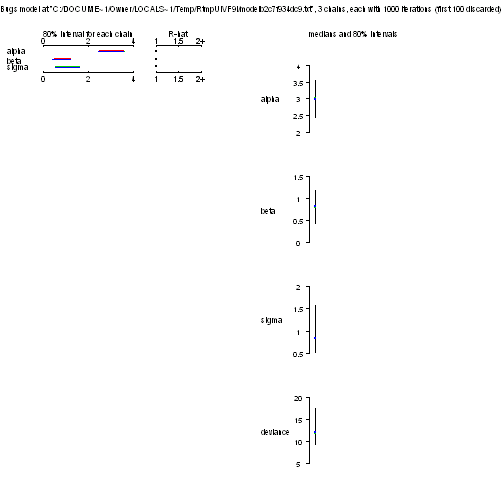The sims.list object pools samples across chains, one for each monitored node. Note that bugs(…) automatically saves the “deviance” as a measure of goodness of fit of the model.

``````names(lineout\$sims.list)
``````
``````##  "alpha"    "beta"     "sigma"    "deviance"
``````

If we wish, we can summarise the quantiles of this object to give other percentiles than the standard used in the summary object. This facilitates calculation of alternative credible intervals.

``````quantile(lineout\$sims.list[], probs = c(0.1, 0.5, 0.9))
``````
``````##   10%   50%   90%
## 2.448 3.005 3.551
``````
``````quantile(lineout\$sims.list\$alpha, probs = c(0.1, 0.5, 0.9))
``````
``````##   10%   50%   90%
## 2.448 3.005 3.551
``````
``````quantile(lineout\$sims.list\$beta, probs = c(0.05, 0.1, 0.2, 0.5, 0.8, 0.9, 0.95,
0.99))
``````
``````##     5%    10%    20%    50%    80%    90%    95%    99%
## 0.2621 0.4272 0.5852 0.8003 1.0230 1.1820 1.3450 1.8013
``````

We can also plot the posterior densities. Normally this is best done using the “coda” package, but a small amount of code will get a similar result.

``````## Density plot without using CODA
plot(density(lineout\$sims.list\$alpha))
abline(v = seq(-1, 6), col = "grey")
abline(v = mean(lineout\$sims.list\$alpha), col = "red", lwd = 1)
abline(v = median(lineout\$sims.list\$alpha), col = "red", lwd = 1, lty = 2)
``````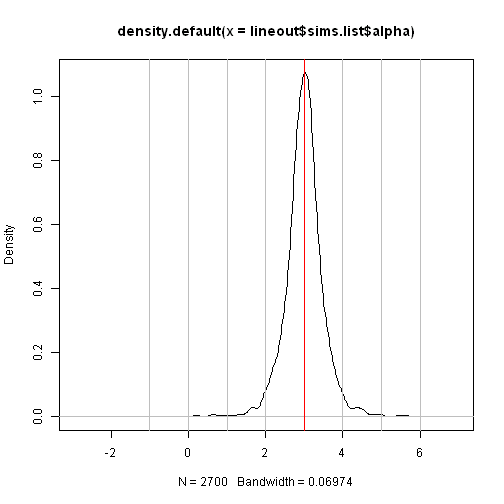``````
plot(density(lineout\$sims.list\$beta))
abline(v = seq(-1, 6), col = "grey")
abline(v = mean(lineout\$sims.list\$beta), col = "red", lwd = 1)
abline(v = median(lineout\$sims.list\$beta), col = "red", lwd = 1, lty = 2)
``````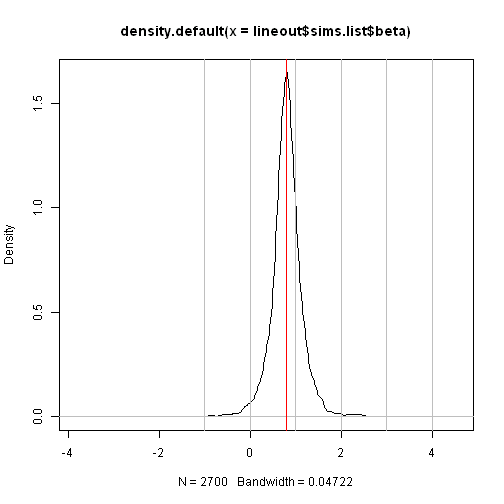``````
plot(density(lineout\$sims.list\$sigma))
abline(v = seq(-1, 6), col = "grey")
abline(v = mean(lineout\$sims.list\$sigma), col = "red", lwd = 1)
abline(v = median(lineout\$sims.list\$sigma), col = "red", lwd = 1, lty = 2)
``````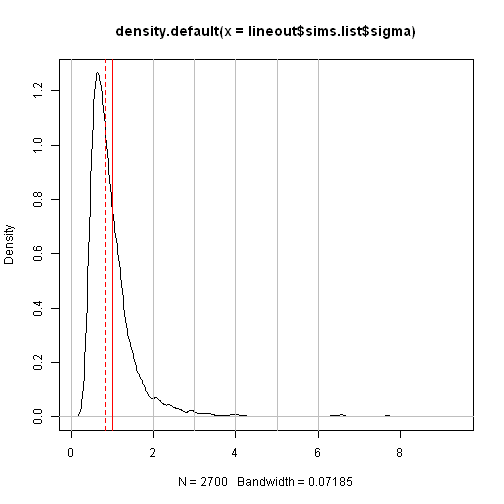The sims.array item contains the MCMC samples for each chain, but is not named. Looking at the dimensions of the array you can see that the array has 900 rows (number of MCMC iterations after the burn-in), 3 columns (MCMC chains) and 4 layers (number of nodes).

``````dim(lineout\$sims.array)
``````
``````##  900   3   4
``````

If you want to produce the MCMC history “Hairy Catepillar” plot then the following code uses the sims.array to produce this. You should be looking for the samples to be centred around a steady mean/median (the catepillar should not wiggle up and down!). Each chain is overlaid in the plot - in black, red and green. Again, the samples from the MCMC should overlay nicely and you shouldn't see noticeably different shapes or average values for the three chains.

``````## 'Hairy catepillar' plots - equivalent to History in BUGS Loop j over
## number of parameters Loop i over number of chains

npars <- length(lineout\$root.short) - 1
for (j in 1:npars) {
main <- paste(lineout\$root.short[j], "MCMC samples")
plot(lineout\$sims.array[, 1, j], type = "n", main = main, ylab = "", ylim = range(lineout\$sims.list[[j]]))

for (i in 1:lineout\$n.chains) {
lines(lineout\$sims.array[, i, j], col = i)
}
}
``````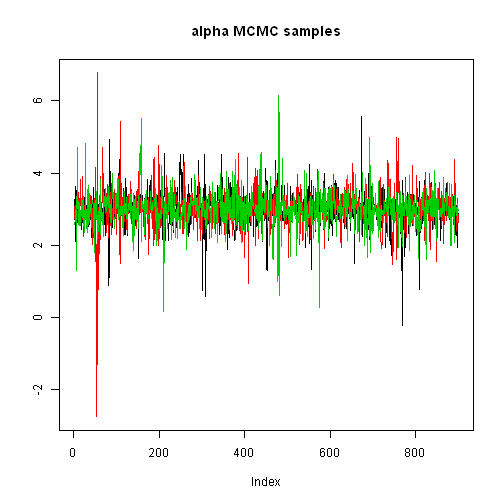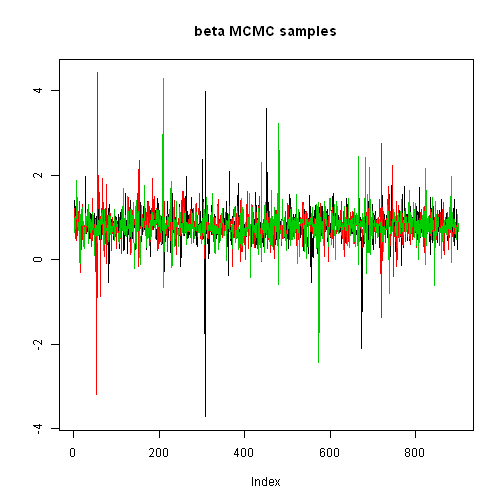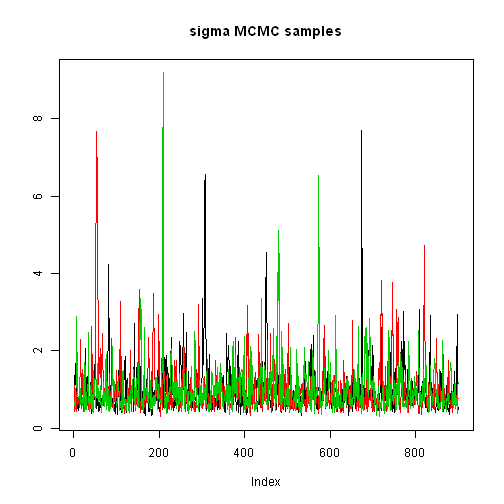Alternative 2

In this version we don't save the individual chain values and only pass back the summary statistics for each node to R. We also choose not to save the DIC/deviance information. This is a good option if you're sure that the MCMC chains are converging easily - as in this case.

``````lineout <- bugs(linedata, lineinits, c("alpha", "beta", "sigma"), linemodel,
n.iter = 1000, n.burnin = 100, n.thin = 1, summary.only = T, DIC = F)

attributes(lineout)
``````
``````## \$names
##  "stats" "DIC"
``````
``````
lineout\$stats
``````
``````##         mean     sd val2.5pc median val97.5pc sample
## alpha 2.9970 0.5292  1.98700 3.0100     3.990   2700
## beta  0.7994 0.3967  0.09888 0.7982     1.514   2700
## sigma 0.9931 0.6595  0.41270 0.8337     2.534   2700
``````

Alternative 3

As complete opposite approach - here we will save out the individual MCMC samples ready for passing to the R package “coda” for assessing convergence, diagnostics, summarising the posterior distributions etc.

``````## Produce CODA output for use with the CODA package
lineout <- bugs(linedata, lineinits, c("alpha", "beta", "sigma"), linemodel,
n.iter = 1000, n.burnin = 100, n.thin = 1, codaPkg = T)

## Need to use CODA to summarise, plot etc.
attributes(lineout)
``````
``````## NULL
``````

Now prepare the lineout object for use with coda

``````library(coda)
``````
``````## Loading required package: lattice
``````
``````## Produce a CODA object from the lineout output
``````
``````## Abstracting alpha ... 900 valid values
## Abstracting beta ... 900 valid values
## Abstracting deviance ... 900 valid values
## Abstracting sigma ... 900 valid values
## Abstracting alpha ... 900 valid values
## Abstracting beta ... 900 valid values
## Abstracting deviance ... 900 valid values
## Abstracting sigma ... 900 valid values
## Abstracting alpha ... 900 valid values
## Abstracting beta ... 900 valid values
## Abstracting deviance ... 900 valid values
## Abstracting sigma ... 900 valid values
``````

First let's look at the summary statistics (as above, but using the coda object)

``````## Summary statistics from the CODA output (uses coda package)
summary(line.coda)
``````
``````##
## Iterations = 101:1000
## Thinning interval = 1
## Number of chains = 3
## Sample size per chain = 900
##
## 1. Empirical mean and standard deviation for each variable,
##    plus standard error of the mean:
##
##            Mean    SD Naive SE Time-series SE
## alpha     2.997 0.529  0.01019        0.01190
## beta      0.799 0.397  0.00764        0.00658
## deviance 12.809 3.563  0.06856        0.13388
## sigma     0.993 0.660  0.01269        0.02593
##
## 2. Quantiles for each variable:
##
##           2.5%    25%    50%    75% 97.5%
## alpha    1.999  2.748  3.005  3.252  3.98
## beta     0.070  0.631  0.800  0.972  1.51
## deviance 8.780 10.190 11.950 14.370 21.62
## sigma    0.415  0.624  0.829  1.143  2.59
``````

Summarising the chains using coda automatically assumes that you want summaries for each chain. If we want to pool samples across chains then we have to do a little manipulation:

``````## to change line.coda to pretend that only 1 chain
line.coda2 <- as.mcmc(as.matrix(line.coda))
``````

We can then summarise across chains:

``````HPDinterval(line.coda2)
``````
``````##            lower  upper
## alpha    1.97900  3.958
## beta     0.06577  1.490
## deviance 8.49400 19.630
## sigma    0.31400  2.059
## attr(,"Probability")
##  0.95
``````

Coda also has many plots for assessing convergence and looking at diagnostics:

``````## Use CODA to produce diagnostic plots for the MCMC
plot(line.coda)
``````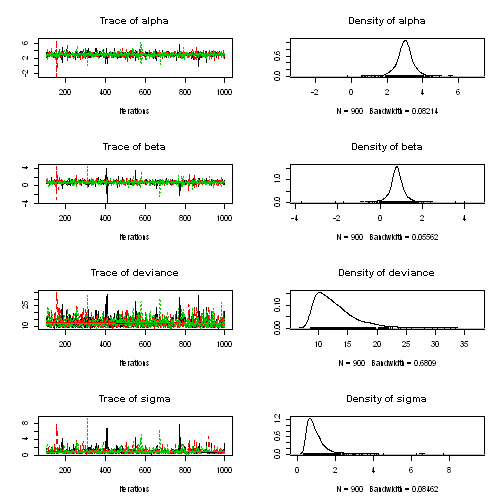``````traceplot(line.coda)
``````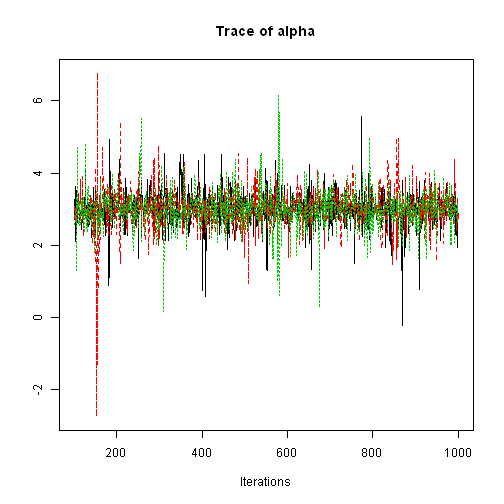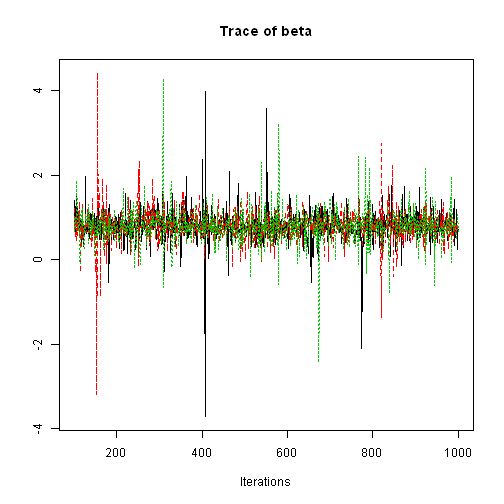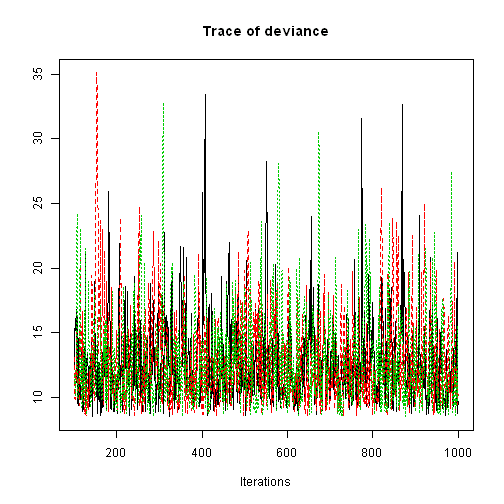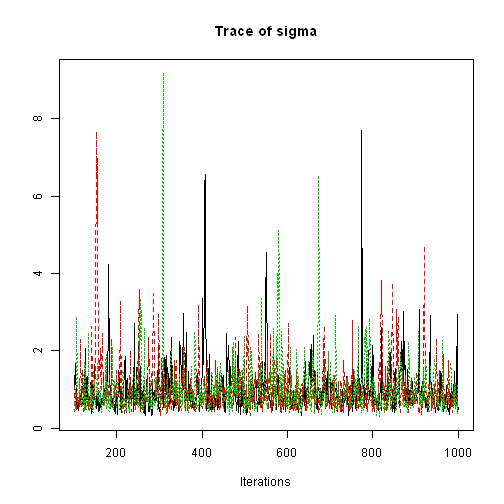``````crosscorr.plot(line.coda)
``````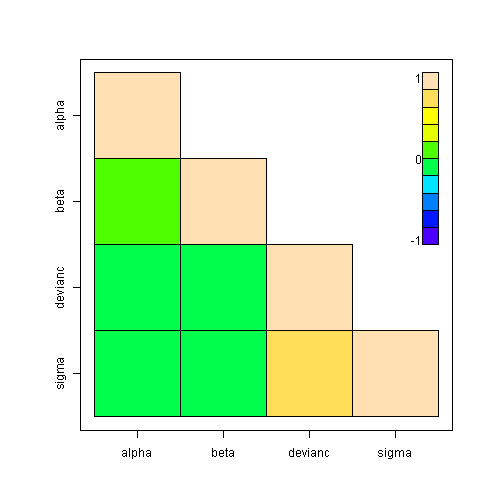You can produce slightly smarter plots using the “lattice” package

``````library(lattice)
xyplot(line.coda)
``````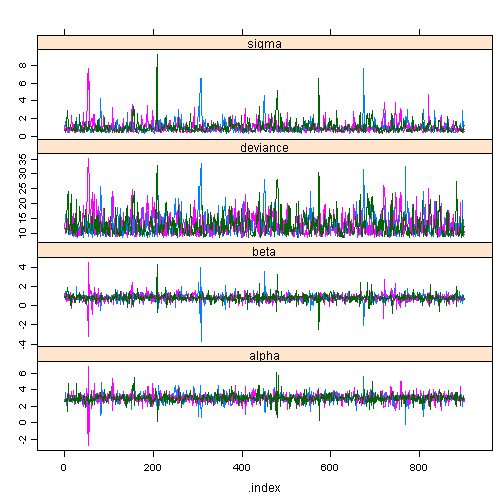``````densityplot(line.coda)
``````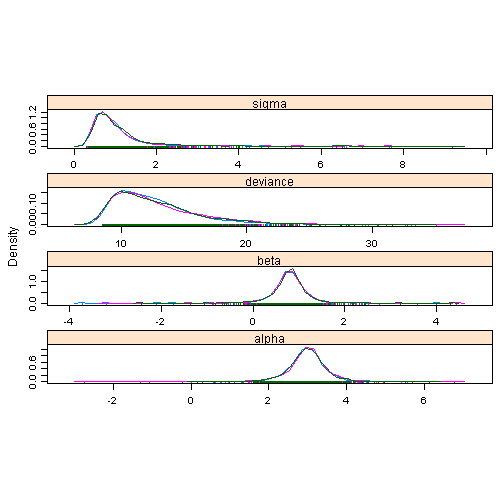A quick sample of some of the other coda diagnostic plots. I'm not going to discuss what these plots tell you, since that would take a lot of extra space! Instead you can find a discussion of many of them across a number of useful references:
coda R package reference
blog on MCMC diagnostics and coda from Florian Hartig
teaching material on MCMC diagnostics by Patrick Lam

``````acfplot(line.coda)
``````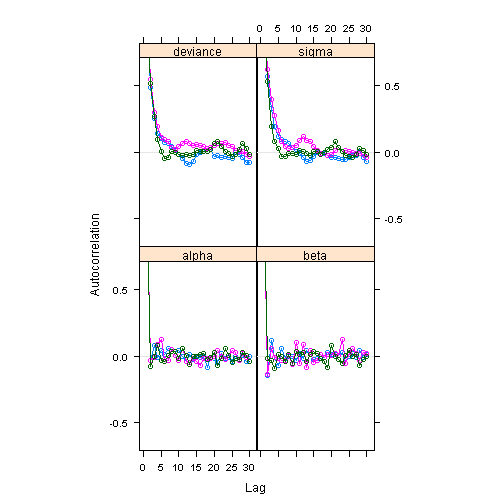``````autocorr.plot(line.coda)
``````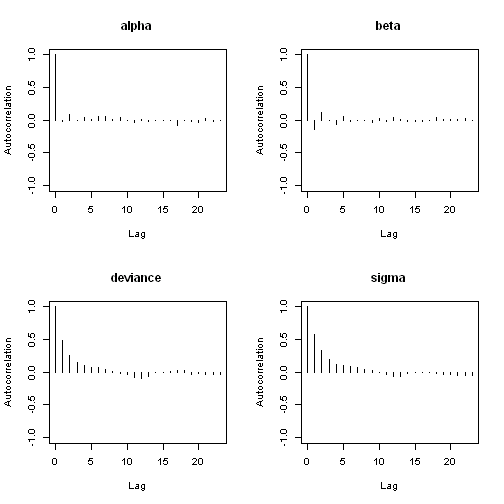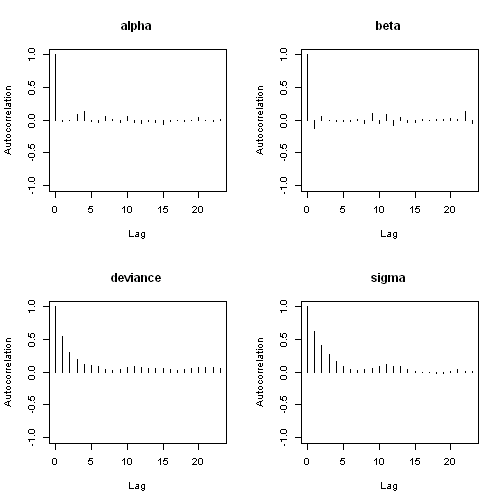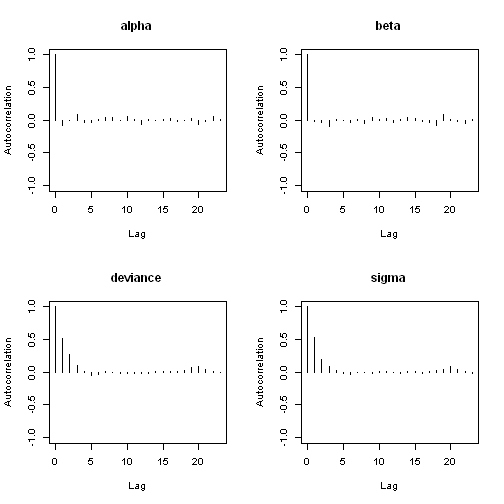``````cumuplot(line.coda)
``````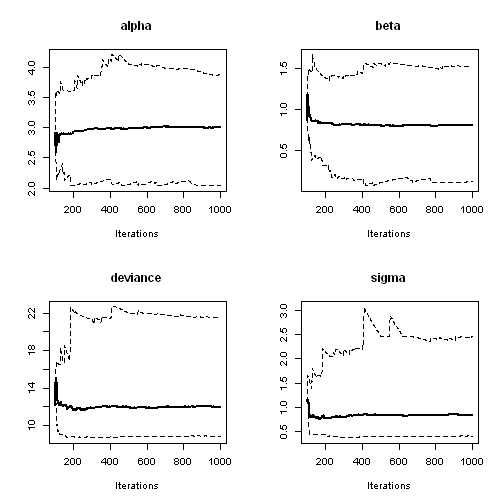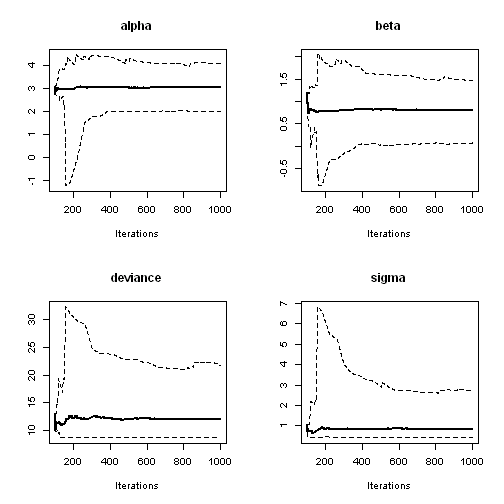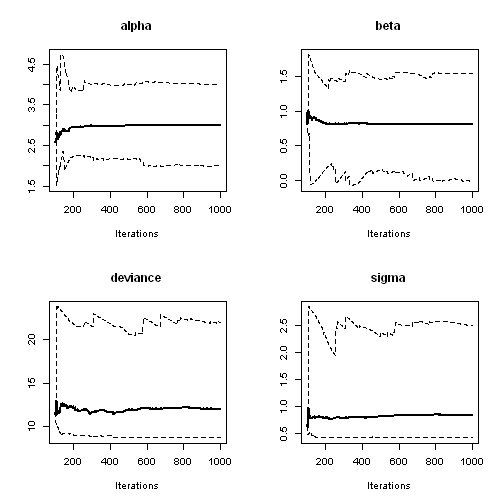``````gelman.plot(line.coda)
``````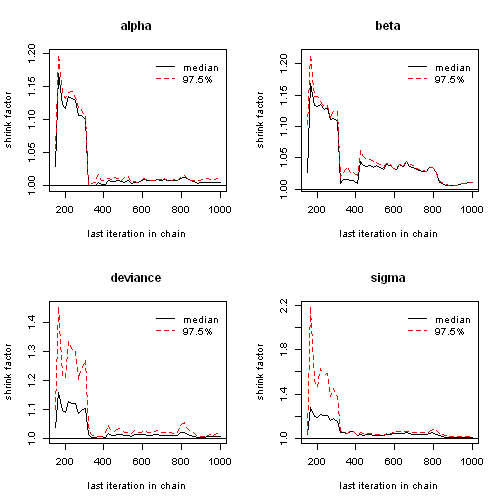``````geweke.plot(line.coda)
``````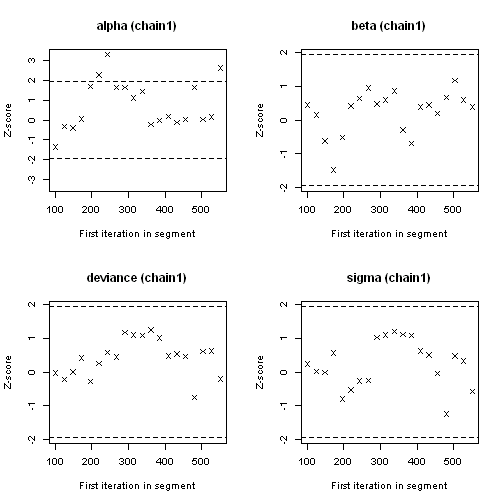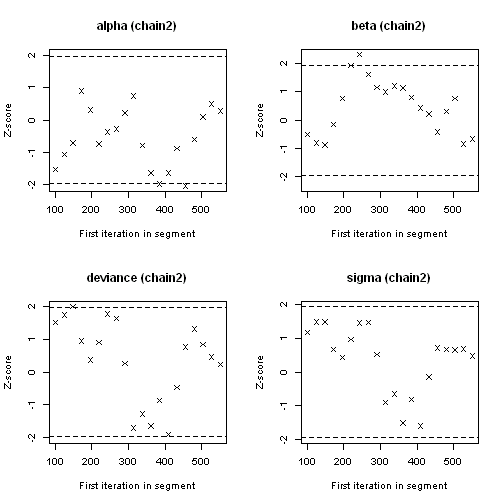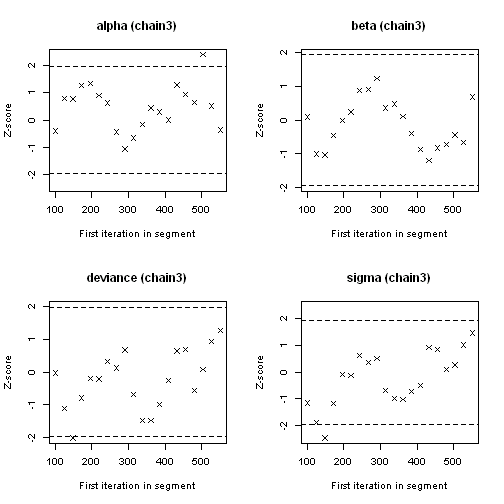Alternative 4

Using R2OpenBUGS it's possible to quite quickly change the model and try something different. Here we add in a prediction into the model (the effect at a new value of x) and then a test on whether the effect at that value of x is > 10. This is effectively the posterior probability P(Effect at X | alpha, beta) > 10. While this is a trivial task for this model, in other cases it's a useful way to get your hands on key inference from your model without having to do any further work. Effectively that inference comes for free as part of the MCMC.

We're also going to change the prior for the precision (1/variance) parameter from a gamma distribution to a half-Normal distribution. This involves setting a bound on the parameter within the model. In BUGS we would just use dnorm(0,1)I(0,) to specify a half-Normal bounded above zero, but here because R is going to parse the model/function object we need to use the syntax dnorm(0,1)`%_%I(0,)`. The `%_%` will be stripped out of the model file when it is passed to BUGS. This prior implies that a priori we expect very low precision (high variance). This is reasonable since we can gain information quite quickly about the magnitude of the residual variance (1/precision).

The step(…) function returns a 1 if the value is greater than or equal to 0, 0 otherwise. We subtract the target value (10) from the pred value and then the posterior mean counts the proportion of samples where the result in brackets is greater than or equal to zero.

``````linemodel2 <- function() {
for (j in 1:N) {
Y[j] ~ dnorm(mu[j], tau)
mu[j] <- alpha + beta * (x[j] - xbar)
}

## Make predictions for a new value of x=10
pred <- alpha + beta * (new.x - xbar)
## Posterior probability that the predicted value is >10 step produces
## value =1 if value is >=0, =0 if <0
pred.10 <- step(pred - 10)
## Posterior prediction interval for new OBSERVATIONS including sigma
Y.new ~ dnorm(pred, tau)

alpha ~ dnorm(0, 0.001)
beta ~ dnorm(0, 0.001)
tau ~ dnorm(0, 1) %_% I(0, )  ## Half-Normal distribution
sigma <- 1/sqrt(tau)
}

linedata <- list(Y = c(1, 3, 3, 3, 5), x = c(1, 2, 3, 4, 5), N = 5, xbar = 3,
new.x = 10)

lineout2 <- bugs(linedata, lineinits, c("alpha", "beta", "sigma", "pred", "pred.10",
"Y.new"), linemodel2, n.iter = 1000, n.burnin = 100, n.thin = 1, summary.only = T,
DIC = F)

lineout2\$stats
``````
``````##           mean     sd val2.5pc    median val97.5pc sample
## Y.new   8.5980 2.9040  3.03100 8.521e+00    14.870   2700
## alpha   2.9950 0.5092  1.99300 2.992e+00     4.061   2700
## beta    0.7990 0.3743  0.06489 7.862e-01     1.563   2700
## pred    8.5880 2.6520  3.44800 8.522e+00    13.930   2700
## pred.10 0.2652 0.4414 -0.08293 1.217e-05     1.020   2700
## sigma   1.0830 0.3969  0.64370 9.851e-01     2.135   2700
``````